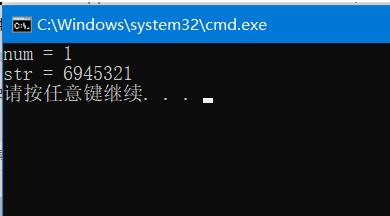• 字符串逆序输出：我先说一个我的错误代码，一开始真的不知道哪里错了，但是当时的想法是自以为是可以的，大家可以先看看我的错误代码这个代码是错误的，正确的在最下方，不要急，我只是展示一下自己的错误，哈哈#...
将字符串逆序输出：我先说一个我的错误代码，一开始真的不知道哪里错了，但是当时的想法是自以为是可以的，大家可以先看看我的错误代码这个代码是错误的，正确的在最下方，不要急，我只是展示一下自己的错误，哈哈#include #include #include #include using namespace std;void reverse(char a[]){char *p=a,*q=a,c;int i;while(*p!='\0')p++;p--;//此刻p指向最后一个元素//  while(p>q)//  {//    c=*p;//    *p=*q;//    *q=c;//    p--;//    q++;//  }i=0;for(i=0; p>=q; i++){a[i]=*(p);//代码就是这里出的错，在下面解释一下printf("*(p)==%c a[%d]==%c\n",*(p),i,a[i]);p--;}}int main(){char a;scanf("%s",a);reverse(a);printf("%s",a);return 0;}上面代码：我一开始想着，定义一个指针p，然后先将指针指向最后一个元素，然后慢慢往回(前面)指，再将指针p指向的值赋给a[i],i从0开始变化，这个想法其实没有错，但是却忽略了，指针只有改变地址的权利，而赋值则是将字符串完全改变了，那么指针一开始指向中间靠后的值的时候，原来a字符串里面中间靠前的字符就会改变(因为是逆着赋值的)，那么到了指针p指向中间靠前的时候，p指的是已经变化过的a中的字符值啦。那么问题来了，怎样做呢，将对称位置的字符交换即可。下面是正确代码，比较简单，不做过多介绍啦#include #include #include #include using namespace std;void reverse(char a[]){char *p=a,*q=a,c;int i;while(*p!='\0')p++;p--;//此刻p指向最后一个元素while(p>q){c=*p;*p=*q;*q=c;//交换对称位置的字符值p--;q++;}}int main(){char a;scanf("%s",a);reverse(a);printf("%s",a);return 0;}补充：C语言 将字符串转化成整数，正序(逆序)输出字符串转化为数字：—‘0'数字转化为字符串：+‘0'#include #include #include #include #include #include void Reverse(char *str)  //逆序{char *p = str;while(*p != '\0')  //找到\0{p++;}p--;  //\0前一位int temp;while(str < p){temp = *str;  //交换*str = *p;*p = temp;str++;p--;}}int Myatoi(const char *str)  //字符串转化为数字{assert(*str != NULL);int sum = 0;while(isdigit(*str)){sum = sum*10+*str-'0';*str++;}return sum;}void Myitoa(char *str,int n)  //数字转化为字符串{assert(*str != NULL);int i = 0;do{str[i++] = n % 10 + '0';n /= 10;}while(n != 0);str[i] = '\0';//Reverse(str);}int main(){char str1 = "1a2";printf("num = %d\n",Myatoi(str1));char str;Myitoa(str,1235496);printf("str = %s\n",str);return 0;}以上为个人经验，希望能给大家一个参考，也希望大家多多支持脚本之家。如有错误或未考虑完全的地方，望不吝赐教。
展开全文• C语言字符串逆序 #include<stdio.h> #include<stdlib.h> #include<string.h> int i, length; int main(){ char input; char rev_input; gets(input); length = strlen(input); ...
 C语言字符串逆序

#include<stdio.h>
#include<stdlib.h>
#include<string.h>
int i, length;

int main(){
char input;
char rev_input;
gets(input);
length = strlen(input);
for(i = 0; i < length; i++){
rev_input[i] = input[length-1-i];
}
printf("%s\n", rev_input);
}


展开全文• 题目描述： 写一函数，使输入的一个字符串按反序存放，在主函数中输入输出反序后的字符串（不包含空格）。 输入： 一行字符 输出： 逆序后的字符串 ... 这道题目要求将输入的字符串逆序输出。首先注意要...
题目描述：
写一函数，使输入的一个字符串按反序存放，在主函数中输入输出反序后的字符串（不包含空格）。
输入：
一行字符
输出：
逆序后的字符串
样例输入：
                             123456abcdef

样例输出：
                             fedcba654321

分析：
   这道题目要求将输入的字符串逆序输出。首先注意要求“不包含空格”，所以不能使用gets()来输入。
然后调用函数，在被调用的函数中先用strlen()函数求出字符串的长度，然后调换字符，使字符串逆
序。最后在主函数中用puts()函数输出。

正确代码：
#include<stdio.h>
#include<string.h>
void change(char a[])
{
int i = 0;
int j = 0;
char t;
j = strlen(a);      //求字符串的长度
for(i = 0;i < j/2;i++)
{
t = a[i];
a[i] = a[j-1-i];
a[j-1-i] = t;
}          //调换对应位置的字符
}
int main()
{
char a;
scanf("%s",a);
change(a);
puts(a);
return 0;
}

知识点：
求字符串的长度用strlen()函数，同时要使用预处理指令#include<string.h>


展开全文• printf("请输入字符：\n"); for(i=0;i;i++) scanf("%c",&a[i]); for(i=0;i;i++) Push(&S,a[i]); for(i=0;i;i++) Pop(&S,&a[i]); for(i=0;i;i++) printf("%c",a[i]); } int InitStack(SqStack *S) {S->base=(char *)...
该楼层疑似违规已被系统折叠 隐藏此楼查看此楼#include"stdio.h"#include"stdlib.h"#define STACK_INIT_SIZE 100#define STACKINCREMENT 10typedef struct{char *base;char *top;int stacksize;}SqStack;main(){SqStack S;char a;int i;InitStack(&S);printf("请输入字符：\n");for(i=0;i<4;i++)scanf("%c",&a[i]);for(i=0;i<4;i++)Push(&S,a[i]);for(i=0;i<4;i++)Pop(&S,&a[i]);for(i=0;i<4;i++)printf("%c",a[i]);}int InitStack(SqStack *S){S->base=(char *)malloc(STACK_INIT_SIZE * sizeof(char));if(!S->base) return 0;S->top=S->base;S->stacksize=STACK_INIT_SIZE;return 1;}int Push(SqStack *S,char e){if(S->top-S->base>=S->stacksize){S->base=(char *)realloc(S->base,(S->stacksize+STACKINCREMENT) * sizeof(char));if(!S->base) return 0;S->top=S->base+S->stacksize;S->stacksize+=STACKINCREMENT;}*S->top++=e;return 1;}int Pop(SqStack *S,char *e){if(S->top==S->base) return 0;*e=*--S->top;return 1;}
展开全文• 【问题描述】将输入的字符串逆序输出 【输入形式】要求输入一个字符串 【输出形式】逆序后输出 【样例输入】abcd 【样例输出】dcba #include <stdio.h> #include<stdlib.h> #include<string.h> ...
• 戆黍熬ｊ纛窦黪鬃用Ｃ语言指针实现字符串逆序及回文串的判定口裴晓英(新疆兵团高等专科学校新疆 鸟鲁木齐８３１３００)摘要：字符串逆序输出Ｃ语言经典算法之一，过去利用数组下标处理此类问题，较为繁琐，本文给...
• *程序功能：字符串逆序输出，用指针 *时间:2019年10月00日 **********************************/ #include<stdio.h> #include<string.h> int main(int argc, const char *argv[])...
• printf("请输入字符：\n"); for(i=0;i;i++) scanf("%c",&a[i]); for(i=0;i;i++) Push(&S,a[i]); for(i=0;i;i++) Pop(&S,&a[i]); for(i=0;i;i++) printf("%c",a[i]); } int InitStack(SqStack *S) {S->base=(char *)...
• 字符串逆序输出：我先说一个我的错误代码，一开始真的不知道哪里错了，但是当时的想法是自以为是可以的，大家可以先看看我的错误代码 这个代码是错误的，正确的在最下方，不要急，我只是展示一下自己的错误，哈哈 ...
• ## c语言字符串逆序总结与辨析

多人点赞 热门讨论 2021-05-20 19:39:43
头文件：#include strrev()函数将字符串逆置，其...因此它只能逆置字符数组，而不能逆置字符串指针指向的字符串，因为字符串指针指向的是字符串常量，常量不能被修改。 【函数示例】看看strrev()是否改变原有字符串
• 头文件 #include <string.h> 函数原型 char *strrev(char *str); 功能 把字符串str的所有字符的顺序颠倒。（逆序） 示例字符串反转 C++
• C语言字符串逆序（一个参数版本+两个参数版本） 一、两个指针参数版本（简单） //添加两个参数 void reverse_string(char* start,char* end){ char tmp = *start; *start = *end; *end = tmp; if (start+1 < ...
• 用不同的方法实现字符串逆序输出(C语言实现)今天去面试遇到面试题如下：请将字符串"123456",逆序输出？当时我使用是指针，我后来想到的其他实现方法。实现如下：#include #include ///////////////////////////用...
• 自己写了一段逆序输出的代码，但是运行没有结果  #include #include int main() { char a; int i; i = 0; while ((a[i]=getchar())!='\r') /*将输入的字符依次保存在a数组中，并递增i，记录字符...
• C语言字符串逆序字符串逆序有多种办法，下面我们分方法而论：//非递归实现字符串反转:char*reverse(char*str){if(!str){returnNULL;}intlen=strlen(str);inti，j;chartemp;for(i=0,j=len-1;i{//交换前后两个...
• 思路：通过将str数组的第1个字符和第n个字符交换位置，第二个和第n-1个交换…实现该功能   方法二： #include #include #include < string .h> void Reverse( char str[], char ptr[]); ...
• #include<stdio.h>#define Max 200int main(){ char str[Max]; int len=0,i; char *strlen; char *left; char temp; printf("输入字符串:"); gets(str); strlen=str; left=str; ...
• 想要实现字符串里的单词逆序输出，如 给定“how are you”，应输出“you are how” 下面的代码想要利用递归算法实现，但发现运行之后没实现，求解！万分感谢！  #include #include void fun(char *p,int i,int ...c strlen 递归算法...

# c语言字符串逆序输出c语言 订阅